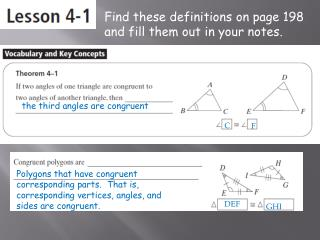# the third angles are congruent - PowerPoint PPT PresentationDownload Presentationthe third angles are congruent

the third angles are congruent
Download Presentation## the third angles are congruent

- - - - - - - - - - - - - - - - - - - - - - - - - - - E N D - - - - - - - - - - - - - - - - - - - - - - - - - - -
##### Presentation Transcript

1. Find these definitions on page 198 and fill them out in your notes. the third angles are congruent C F Polygons that have congruent corresponding parts. That is, corresponding vertices, angles, and sides are congruent. DEF GHI

2. When you name congruent polygons, always list corresponding vertices in the same order. T C BC QJ

3. Read through the example on the top of page 199 and then fill in the blanks below. 180 m<M 48 48 67 115 65

4. Proving Triangles Congruent • We are able to conclude that two triangles are congruent if their corresponding sides and corresponding angles are congruent to each other.

5. Now read through Example 3 on pg. 199 <DCE <DEC Is NOT DE DC EC <DEC <CDE <ACB ABC EDC

6. Oh YEAH? Prove IT! Given • Reflexive Given Definition of perpendicular lines. All right angles congruent. <CGN congruent to <DGN congruent. 4-1 congruent.

7. On Your Own for this one: Given Theorem 4-1 – If 2 angles are congruent in 2 triangles, then their 3rd angles are congruent. <ABE congruent to <DBC Given Definition of congruent triangles

8. Review

9. Review

10. Homework  • Geometry 1st and 3rd period • Practice 4.1, pg. 201, 16, 18, 32, 34 • Honors Geometry 5th period • Practice 4.1, pg 201, 16, 18, 32, 34, 44